# Radix point facts for kids

Kids Encyclopedia Facts

In mathematics and computing, a radix point (or radix character) is a symbol used to separate whole numbers (integers) from fractions. For example, the number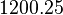$1200.25$ represents an integer of 1200 with a fractional part of 25, and these are separated with a decimal point.

Radix point is the umbrella term for this point in all bases. The most commonly known example is the decimal point, named so for its use in base 10 notation. Similarly, "binary point" is used for base 2. In most English-speaking countries the radix point is usually a small dot (.), but this can vary as other languages can use different notation such as a comma (,) instead.

## Examples

In mathematical notation, each column of numbers represents a power of the radix, with a radix point separating negative powers. For example, the base 10 number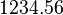$1234.56$ is read as follows:

 Powers Value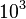$10^3$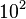$10^2$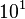$10^1$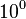$10^0$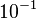$10^{-1}$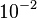$10^{-2}$ 1 2 3 4 5 6

Hence, we can unwrap the representation as this: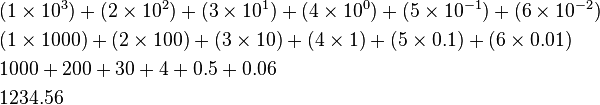\begin{align} &(1 \times 10^3) + (2 \times 10^2) + (3 \times 10^1) + (4 \times 10^0) + (5 \times 10^{-1}) + (6 \times 10^{-2})\\ &(1 \times 1000) + (2 \times 100) + (3 \times 10) + (4 \times 1) + (5 \times 0.1) + (6 \times 0.01)\\ &1000 + 200 + 30 + 4 + 0.5 + 0.06 \\ &1234.56 \end{align}

To the left of the radix point is the integer parts (made from positive powers of base 10). To the right of the radix point is the fractional parts (made from negative powers).

## Related pagesRadix point Facts for Kids. Kiddle Encyclopedia.